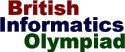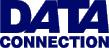The British Informatics Olympiad is the computing competition for schools and colleges. We are proud to present BIO'99.The 1999 British Informatics Olympiad - Round One

 Question 3 Playing games Romulus is playing a game which is divided into rounds. At the end of each round points are awarded depending on the result, and at the end of the game these points are summed for the final score.Given a final score, Romulus is interested in finding the minimum number of rounds that might have taken place. For example: 3 or 5 points are awarded at the end of each round, and Romulus finished with a score of 15. The minimum number of rounds is 3 (each scoring 5). Note that some scores, such as 4, are impossible to obtain in this case.
 3 (a) [26 marks] Write a program that inputs a list of possible points that can be awarded in a round, followed by a list of final scores. For each final score you should output the minimum number of rounds that might have taken place, along with the corresponding points scored.The first line of input will consist of a single integer n (1<=n<=10) denoting the number of possible points that can be scored in a round. The second line will consist of n integers (between 1 and 500) giving the possible points. Note that the numbers in the second line will not necessarily be sorted. The third line of input will be a single integer m (1<=m<=10) indicating the number of final scores to be considered. The fourth line will give the m final scores to be considered (between 1 and 1,000). Your output should consist of m lines, one for each of the final scores. Each line should contain the minimum number of rounds, followed by a corresponding example set of points. You should follow the format given in the sample run; note that the example set does not need to be sorted. If it is not possible to produce a given score you should just output Impossible on that line. Sample run 6 50 10 2 5 1 20 3 10 49 101 1 1x10 5 1x5 2x20 2x2 3 2x50 1x1
 3 (b) [4 marks] Romulus is playing a game where it is possible to score 1, 3, 9, 27 or 81, and after he is finished Remus also plays the same game. When they compare their final scores Romulus has scored 80 more than Remus. What is the minimum number of rounds they may have played between them? How about if Romulus has 50 more points than Remus? [Give an example in each case.] 3 (c) [5 marks] Remus is playing a game where it is possible to score 1, 4, 5, 17, 28, 43 or 100 each round. At the end of the game the final score is 100. Furthermore, the scores for each round never got worse, e.g. if 17 was scored in one round then the score for every future round was at least 17. How many different ways might this have happened?